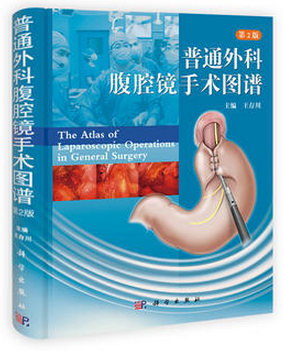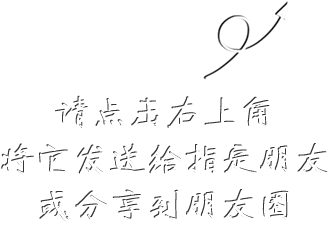# 普通外科腹腔镜手术图谱（第2版）

## ¥ 238.40已售出89件

《普通外科腹腔镜手术图谱（第2版）》根据作者20多年来开展腹腔镜外科的经验编撰而成，每种手术自成一章，共91章，侧重于比较常见、比较复杂的一些普通外科腹腔镜手术，特别是胃肠道、肝、胆、胰、脾、甲状腺、乳腺、疝、肥胖与代谢病手术。全书配有近2000幅彩色的手术过程图片，以图片为主，文字为辅，介绍了开展手术所需要的仪器设备、手术适应证、手术禁忌证、手术操作方法和围手术期处理等，详细介绍了肝、胆、胰、脾、胃、肠、疝、甲状腺、乳腺、血管等腹腔镜手术方法，内容新颖，以帮助读者了解普通外科腹腔镜手术的基本知识和手术方法。《普通外科腹腔镜手术图谱（第2版）》适合于已经开展腹腔镜手术及准备开展腹腔镜手术的各级普通外科医生阅读。

• 删除
• 删除
• 删除
• 删除
• 删除
• 删除
• 删除
• 删除
• 删除
• 删除
• 删除
• 删除
• 删除
• 删除
• 删除
• 删除
• 删除
• 删除
• 删除
• 删除
• 删除
• 删除
• 删除
• 删除
• 删除
• 删除
• 删除
• 删除
• 删除
• 删除
• 删除
• 删除
• 删除
• 删除
• 删除
• 删除
• 删除
• 删除
• 删除
• 删除
• 删除
• 删除
• 删除
• 删除
• 删除
• 删除
• 删除
• 删除
• 删除
• 删除
• 删除
• 删除
• 删除
• 删除
• 删除
• 删除
• 删除
• 删除
• 删除
• 删除
• 删除
• 删除
• 删除
• 删除
• 删除
• 删除
• 删除
• 删除
• 删除
• 删除
• 删除
• 删除
• 删除
• 删除
• 删除
• 删除
• 删除
• 删除
• 删除
• 删除
• 删除
• 删除
• 删除
• 删除
• 删除
• 删除
• 删除
• 删除
• 删除
• 删除
• 删除
• 删除
• 删除
• 删除
• 删除
• 删除
• 删除
• 删除
• 删除
• 删除
• 删除
• 删除
• 删除
• 删除
• 删除
• 删除
• 删除
• 删除
• 删除
• 删除
• 删除
• 删除
• 删除
• 删除
• 删除
• 删除
• 删除
• 删除
• 删除
• 删除
• 删除
• 删除
• 删除
• 删除
• 删除
• 删除
• 删除
• 删除
• 删除
• 删除
• 删除
• 删除
• 删除
• 删除
• 删除
• 删除
• 删除
• 删除
• 删除
• 删除
• 删除
• 删除
• 删除
• 删除
• 删除
• 删除
• 删除
• 删除
• 删除
• 删除
• 删除
• 删除
• 删除
• 删除
• 删除
• 删除
• 删除
• 删除
• 删除
• 删除
• 删除
• 删除
• 删除
• 删除
• 删除
• 删除
• 删除
• 删除
• 删除
• 删除
• 删除
• 删除
• 删除
• 删除
• 删除
• 删除
• 删除
• 删除
• 删除
• 删除
• 删除
• 删除
• 删除
• 删除
• 删除
• 删除
• 删除
• 删除
• 删除
• 删除
• 删除
• 删除
• 删除
• 删除